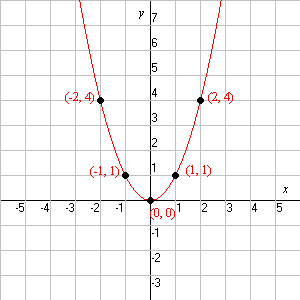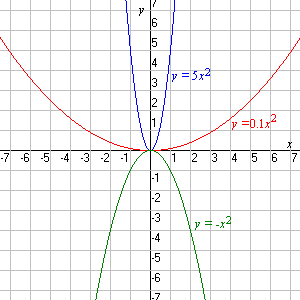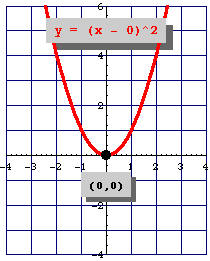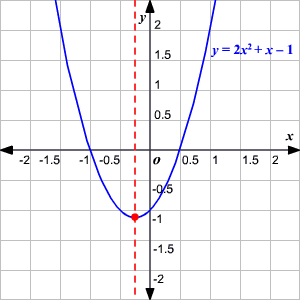The general form of a quadratic function is $f\left(x\right)=a{x}^{2}+bx+c$ . The graph of a quadratic function is a parabola , a type of $2$ -dimensional curve.

The "basic" parabola, $y={x}^{2}$ , looks like this:The function of the coefficient $a$ in the general equation is to make the parabola "wider" or "skinnier", or to turn it upside down (if negative):If the coefficient of ${x}^{2}$ is positive, the parabola opens up; otherwise it opens down.

## The Vertex

The vertex of a parabola is the point at the bottom of the " $\text{U}$ " shape (or the top, if the parabola opens downward).

The equation for a parabola can also be written in "vertex form":

$y=a{\left(x-h\right)}^{2}+k$

In this equation, the vertex of the parabola is the point $\left(h,k\right)$ .You can see how this relates to the standard equation by multiplying it out:

$y=a\left(x-h\right)\left(x-h\right)+k$

$y=a{x}^{2}-2ahx+a{h}^{2}+k$

The coefficient of $x$ here is $-2ah$ . This means that in the standard form, $y=a{x}^{2}+bx+c$ , the expression

$-\frac{b}{2a}$

gives the $x$ -coordinate of the vertex.

Example:

Find the vertex of the parabola.

$y=3{x}^{2}+12x-12$

Here, $a=3$ and $b=12$ . So, the $x$ -coordinate of the vertex is:

$-\frac{12}{2\left(3\right)}=-2$

Substituting in the original equation to get the $y$ -coordinate, we get:

$y=3{\left(-2\right)}^{2}+12\left(-2\right)-12$

$=-24$

So, the vertex of the parabola is at $\left(-2,-24\right)$ .

## The Axis of Symmetry

The axis of symmetry of a parabola is the vertical line through the vertex. For a parabola in standard form, $y=a{x}^{2}+bx+c$ , the axis of symmetry has the equation

$x=-\frac{b}{2a}$

Note that $-\frac{b}{2a}$ is also the $x$ -coordinate of the vertex of the parabola.

Example:

Find the axis of symmetry.

$y=2{x}^{2}+x-1$

Here, $a=2\text{\hspace{0.17em}}\text{\hspace{0.17em}}\text{and}\text{\hspace{0.17em}}\text{\hspace{0.17em}}b=1$ . So, the axis of symmetry is the vertical line

$x=-\frac{1}{4}$## Intercepts

You can find the $y$ -intercept of a parabola simply by entering $0$ for $x$ . If the equation is in standard form, then you can just take $c$ as the $y$ -intercept. For instance, in the above example:

$y=2{\left(0\right)}^{2}+\left(0\right)-1=-1$

So the $y$ -intercept is $-1$ .

The $x$ -intercepts are a bit trickier. You can use factoring , or completing the square , or the quadratic formula to find these (if they exist!).

## Domain and Range

As with any function, the domain of a quadratic function $f\left(x\right)$ is the set of $x$ -values for which the function is defined, and the range is the set of all the output values (values of $f$ ).

Quadratic functions generally have the whole real line as their domain: any $x$ is a legitimate input. The range is restricted to those points greater than or equal to the $y$ -coordinate of the vertex (or less than or equal to, depending on whether the parabola opens up or down).